# RD Sharma Solutions for Class 9 Maths Chapter 12 Heron's Formula Exercise VSAQs

RD Sharma solutions for class 9 Maths provides the exercise VSAQs problems of the chapter “Heron’s Formula”. With these detailed solutions, the students will be able to clear all their confusion and able to find the area of any triangle whose sides are known using heron’s formula. Find detailed RD Sharma class 9 solutions for chapter 12 Maths here and clear all your doubts.

## Download PDF of RD Sharma Solutions for Class 9 Maths Chapter 12 Heron’s Formula Exercise VSAQs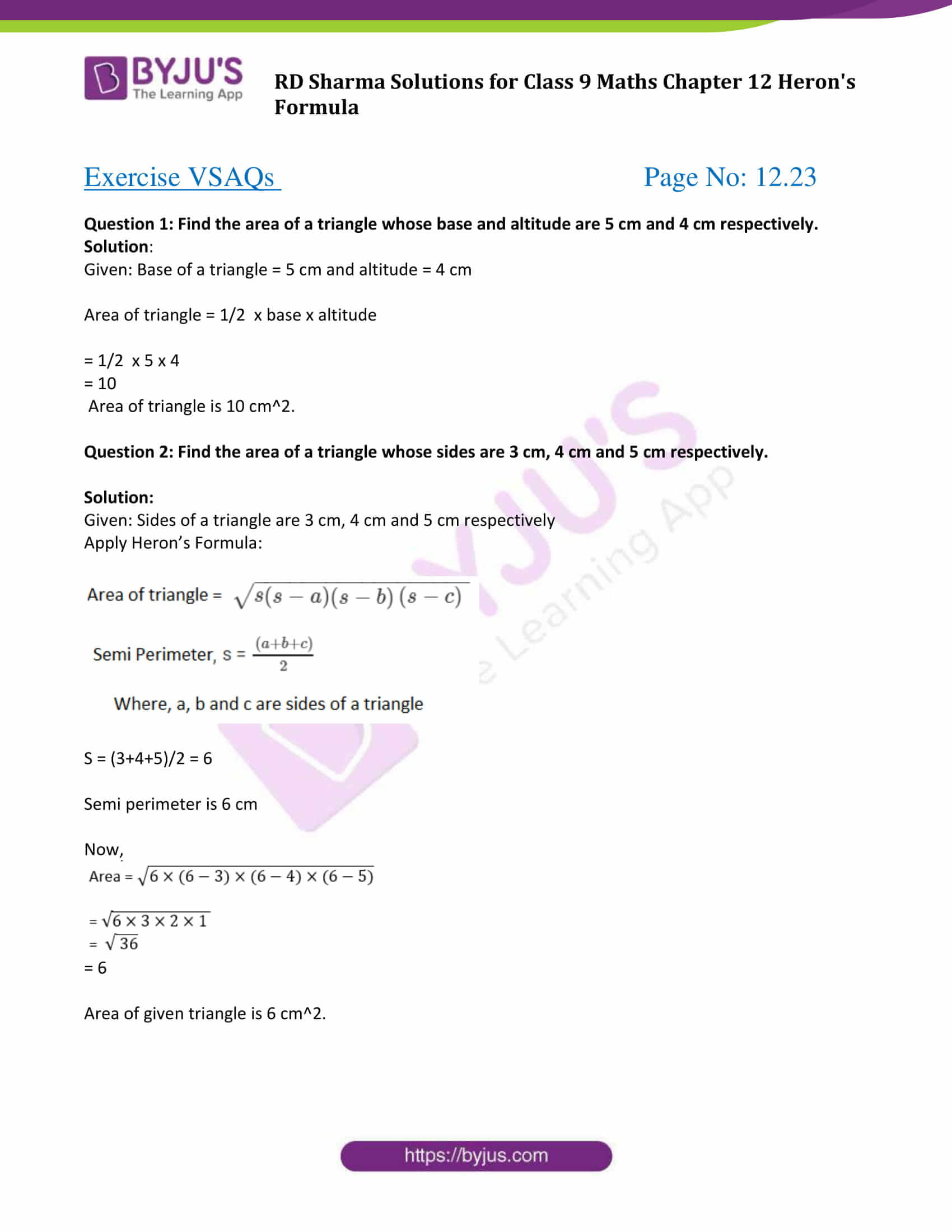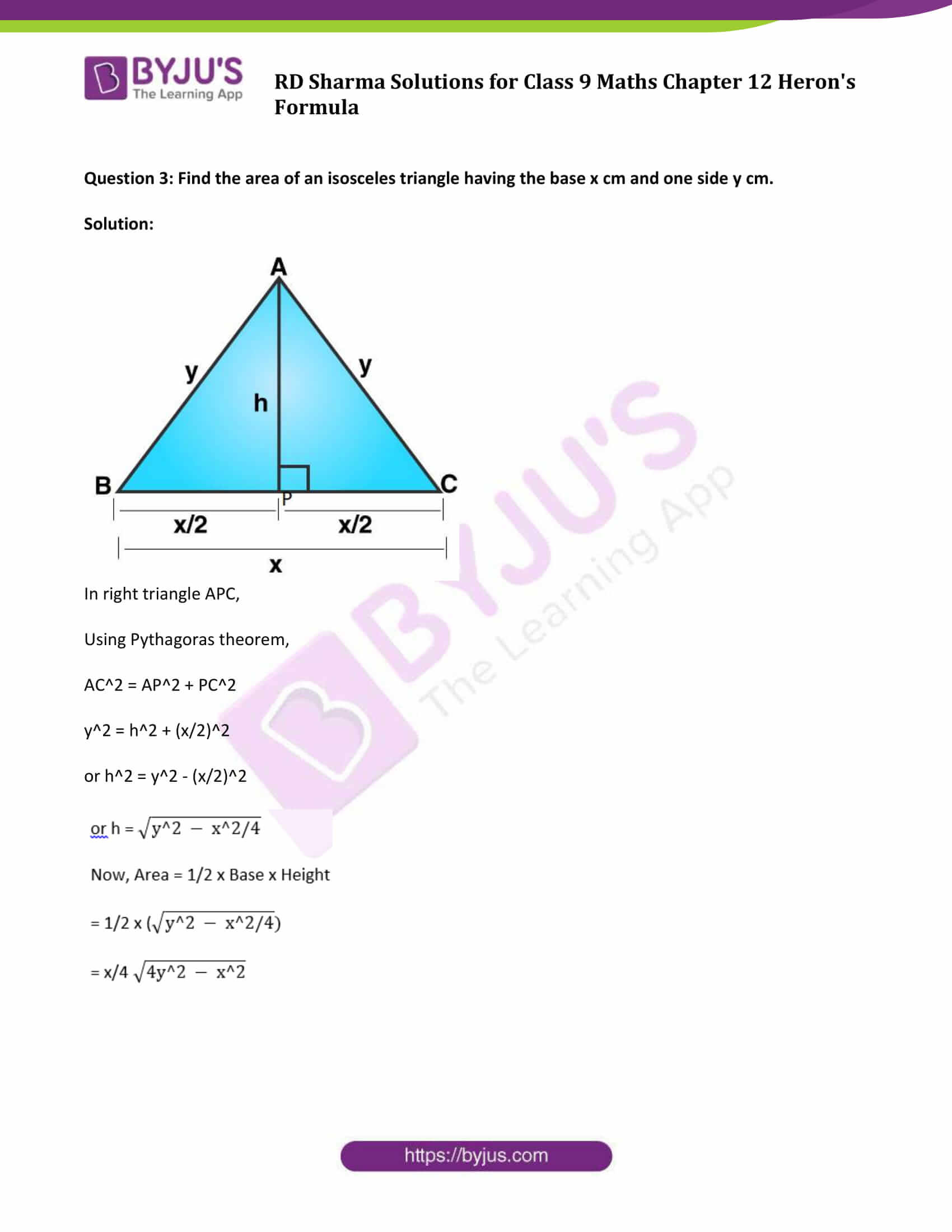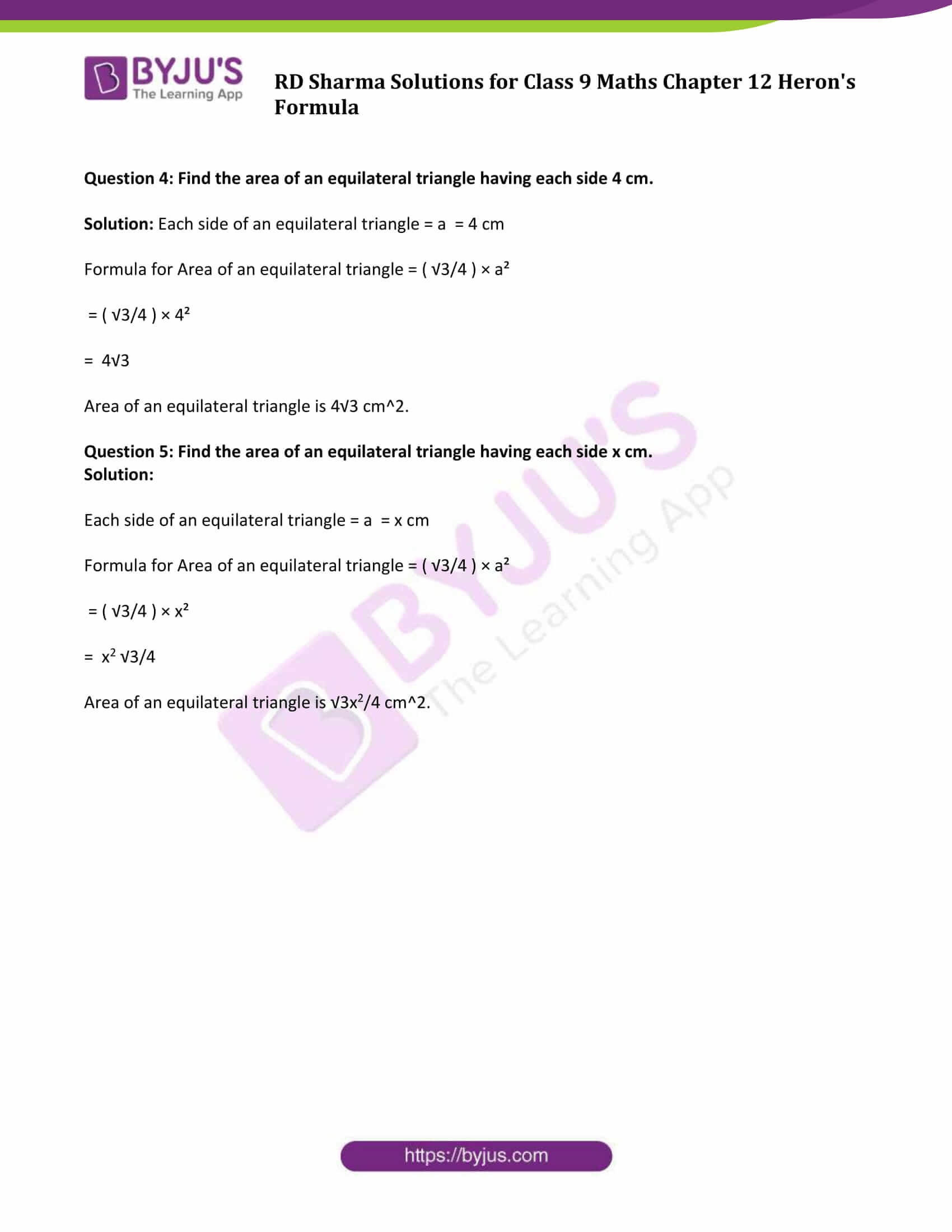### Access Answers to Maths RD Sharma Class 9 Chapter 12 Heron’s Formula Exercise VSAQs Page number 12.23

Question 1: Find the area of a triangle whose base and altitude are 5 cm and 4 cm respectively.

Solution:

Given: Base of a triangle = 5 cm and altitude = 4 cm

Area of triangle = 1/2 x base x altitude

= 1/2 x 5 x 4

= 10

Area of triangle is 10 cm2.

Question 2: Find the area of a triangle whose sides are 3 cm, 4 cm and 5 cm respectively.

Solution:

Given: Sides of a triangle are 3 cm, 4 cm and 5 cm respectively

Apply Heron’s Formula: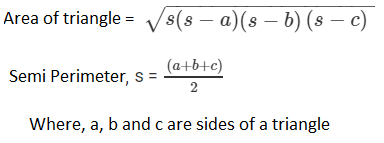S = (3+4+5)/2 = 6

Semi perimeter is 6 cm

Now,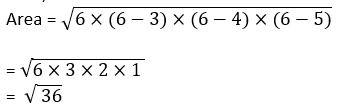= 6

Area of given triangle is 6 cm2.

Question 3: Find the area of an isosceles triangle having the base x cm and one side y cm.

Solution: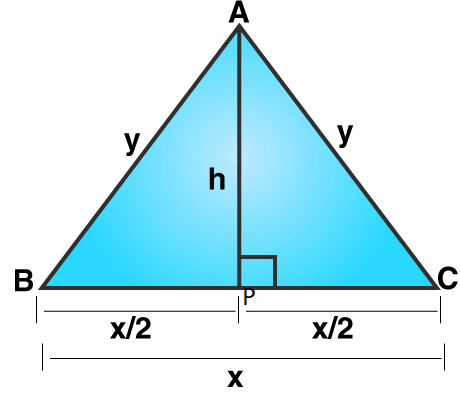In right triangle APC,

Using Pythagoras theorem,

AC2 = AP2 + PC2

y2 = h2 + (x/2)2

or h2 = y2 – (x/2)2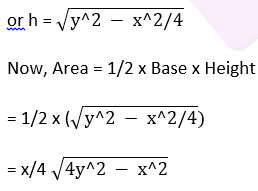Question 4: Find the area of an equilateral triangle having each side 4 cm.

Solution: Each side of an equilateral triangle = a = 4 cm

Formula for Area of an equilateral triangle = ( √3/4 ) × a²

= ( √3/4 ) × 4²

= 4√3

Area of an equilateral triangle is 4√3 cm2.

Question 5: Find the area of an equilateral triangle having each side x cm.

Solution:

Each side of an equilateral triangle = a = x cm

Formula for Area of an equilateral triangle = ( √3/4 ) × a²

= ( √3/4 ) × x²

= x2 √3/4

Area of an equilateral triangle is √3x2/4 cm2.

## RD Sharma Solutions for Class 9 Maths Chapter 12 Exercise VSAQs

RD Sharma Solutions Class 9 Maths Chapter 12 Heron’s Formula Exercise VSAQs is based on Heron’s formula and its applications. Learn this topic in depth with the RD Sharma Class 9 Solutions with step by step illustrations, which will make learning easy for students.